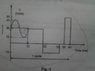# Average value of current from graph.

• tasnim rahman

#### tasnim rahman

This isn't exactly homework, for which I am not posting in the homework section. I am currently doing my undergrads, and am in the second semester of my first year. I am going to give my finals which start from tomorrow, with the first subject being Electrical Circuits II. I was solving the past papers and got stuck on one of the questions, as shown in the photo. In this question, they asked to determine the average value of the current over 1 cycle of it. Please ignore the line drawn through the first oscillatory part. I had mistakenly drawn it with pen, while attempting to solve it. It does not exist there. While it is easy to solve for the average value for any other part of the curve, the problem lies with the first oscillatory part. It consists of two separate sine waves, the first and second quadratures of which have been joined together, one with a maximum/minimum of 10 and the other with a maximum/minimum of 20. I do not know how to find the average value for this part. I tried to solve it, by taking two separate equations for the two sine wave sections and then integrating over them with the respective limits. Like this: ∫(sin wave 1) dt (limits 6 to 0) + ∫ (sin wave 2) dt (limits 12 to 6). But I can't be sure of the limits as there is no logic behind choosing them as such. And also I am not sure if this is the right way. Please, somebody help me find out the average value of the curve, as soon as possible. Thanks in advance.

#### Attachments

•Photo0517.jpg
20.8 KB · Views: 744

I assume you mean average value of volts.

I don't see what you are talking about in the "first osc part". I just see 1 cycle of a sine wave. The average voltage of a sine wave over 1 cycle is zero. So just average everything as if the sine wave is not there.

I am sorry, but you probably didn't see the "sine wave" thoroughly enough. Yes, it starts and ends at 30, as if representing a cycle. But, if you notice the peaks you will see, one goes from 30 to 40, representing a change of +10, while the other goes from 30 to 10, representing a change of -20. Clearly, implying sections of two different sine waves, as if joined together. And this is precisely the problem. It would be very helpful, if you would see the curve again.

Could someone kindly help me solve this problem. Its just that I am in a hurry, because I have an exam tomorrow on this.

Last edited:
X avg = (1/τ) ∫x(t)dt from 0 to τ

Integrate positive and negative side separately then average the two together.

I know that much, thank you. The problem is with the "sine wave". If you would read my previous post, the one before the last post, you would see that it is one continuous sine wave, but more like two sine waves joined up. How do I find the average value for that part. @ psparky.

Last edited:
I know that much, thank you. The problem is with the "sine wave". If you would read my previous post, the one before the last post, you would see that it is one continuous sine wave, but more like two sine waves joined up. How do I find the average value for that part. @ psparky.

He did read your post. First, the graph's vertical axis is very misleading. It is not linear and it is not logarithmic. Poorly drawn, IMO.

So just integrate the first half-cycle of the sine wave using a function with an offset and the sine amplitude as shown on the graph. Then integrate the 2nd half-cycle using the offset and amplitude shown on the graph. Then add in the other parts and divide by the total time.

I know that much, thank you. The problem is with the "sine wave". If you would read my previous post, the one before the last post, you would see that it is one continuous sine wave, but more like two sine waves joined up. How do I find the average value for that part. @ psparky.

Assuming the period is 12...or 6 for one half of the wave.

Don't you just integrate from 0 to 6 ∫10sin(∏/6) then multiply by (1/12)
(the amplitude of 10 is obtained from 40-30=10)

Then integrate from 6 to 12 ∫20sin(∏/6) then multiply by (1/12)
(the amplitude of 20 is obtained from 30-10=20)

Add two results together and divide by two then add or subtract from your original 30 axis...subtract in this case.

Again, I am assuming the period "splits" at 6.

Last edited: